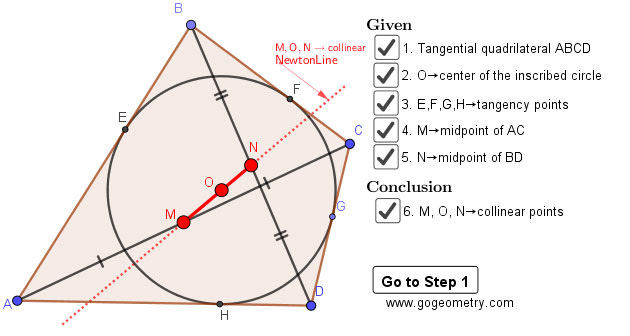## Thursday, March 26, 2020

### Geometry Problem 1466: Tangential Quadrilateral, Newton Line, Incenter, Midpoint, Diagonal, Collinear Points

Interactive step-by-step animation using GeoGebra. Post your solution in the comment box below.
Level: Mathematics Education, High School, Honors Geometry, College.

Details: Click on the figure below.1.From midpoint of BC draw a line through O to AD, the same from midpoint of CD
Two lines meet AD and AB to their midpoints (use tangent segments, equal right triangles). According to P1462 (M4) is point O (complete the figure if necessary). So M, O, N are collinear

2.Join EH,EF,FG and GH, let their midpoints be A',B',C' and D' respectively.
We have OA.OA'=OB.OB'=OC.OC'=OD.OD'=r^2. Hence Quad. A'ACC' and Quad.B'BDD' are cyclic. Tr.OAC is similar to Tr.OC'A', since OM is median for triangle OAC, it is symmedian of Tr.OC'A' and similary ON is symmedian for Tr.OB'D'.

Let A'C' and B'D' meet at point P, then P is mid point of A'C' and B'D'.
OP is median for both Tr.OA'C' and OB'D'. Let Angle POC'=x and Angle POD'=y
Then Angle C'OD'= x+y=180-Angle FGH
We get x+y+G=180
Since OM and ON are symmedians, we have Angle MOA'=x and Angle NOB'=y.
Angle A'OB'=180-E=G .
Angle MON = x+y+G=180, hence M,O and N are collinear.